# Simulation Study of a Flat Plate Solar Collector Influence of the Mixture Air Flow

Simulation Study of a Flat Plate Solar Collector Influence of the Mixture Air Flow
Laboratoire MECACOMP, University of Abou Bekr Bekaid Tlemcen, Tlemcen 13100, Algeria

University of Tlemcen, Bp 230 Chetouane Tlemcen, Algeria

Corresponding Author Email:
sichaibamel@yahoo.com
Page:
12-16
|
DOI:
https://doi.org/10.18280/psees.050103
10 September 2020
|
Accepted:
12 October 2021
|
Published:
31 December 2021
| Citation

OPEN ACCESS

Abstract:

Due to their simple composition, flat plate solar collectors are constantly applied to various fields. As a result, thermo-fluid performance is continuously improving. The direct heat losses in a flat plate solar collector depend essentially on the absorber-glazing clearance. The increase in this clearance leads to an increase in convective losses and its reduction reduces the air flow to be transported. A solution is envisaged by the present subject, which consists in carrying out a new design of an absorber for those with baffles or part of the heat transfer fluid circulating inside. The work consists in optimizing the annular clearance or in circulating the heat transfer fluid because this clearance is made to the detriment of the thickness of the absorber, source of thermal energy of the solar collector. The optimum distance is then sought, which ensures a homogeneous mixture between the current of the main fluid flowing between the baffles on the one hand and the current of the secondary fluid flows in the annular clearance of the absorber on the other hand.

Keywords:

numerical simulation, type of absorber, heat transfer, solar collector, baffle

1. Introduction

The demand for energy in the world is increasing rapidly due to population explosion and technological progress. For this, it is important for humanity to turn to a reliable source of energy based on these two characteristics that is cost effective and a permanent source of renewable energy for future energy demand.

Solar energy is one of the renewable energy sources and is a promising source to solve the problems related to the energy crisis in the future.

We have synthesized and developed a methodology which makes it possible to study air plane solar collectors and in particular those using air as heat transfer fluid. For more precision and rigor, all the elements which make it possible to define knowledge models of planar solar collectors, have been reported with all the details necessary for their integration in processes such as: modeling, complete simulation, ...

Bemar al.  have shown that for a laminar flow baffle channel, Reynolds is less than 600.

Cheng and Huang  studied forced convection between two parallel flat plates provided with transverse fins (transverse baffles) which are not placed symmetrically. Their results indicated that the relative position of the rows of baffles is an influencing factor on the flow field, especially for large baffles.

Hwang et al.  also analyzed laminar flows, forced convection in the entrance region of a horizontal channel. The calculation of the semi-infinite channel in which one or two pairs of baffles are symmetrically fixed to the respective walls in the entry region has been analyzed.

Saim et al.  presented a numerical study of the turbulent flow in a pipe containing a barrier. Numerical results show that the extension of the recycling zone upstream of the obstacle does not depend on its length in the direction of flow. The recirculation zone is strongly influenced by the length of the obstacle; this area decreases as the length of the barrier increases.

Aliane and Amraoui  presented a numerical study of the dynamic and thermal behavior of a turbulent air flow in a horizontal channel of rectangular section provided with transverse baffles. The numerical results obtained indicate that the profiles and the distribution of the axial velocity show a relatively intense recirculation zone above the facets of each baffle which moves downstream. The highest disturbance is obtained upstream of the second deflector. These vortices are responsible for local variations in the Nusselt number along the surfaces of the partitions and in particular those located on the lower side.

Youcef-Ali  have made a numerical study using the finite volume method on the influence of roughness at the level of the insulation. It is noted that the velocities are low for the two types of collectors studied, at the level of the roughness this velocity becomes almost zero. Concerning the temperature fields, and for the case without roughness, the temperature becomes significant just downstream of the second deflector. On the other hand, in the case of roughness, the temperatures assume significant and progressive values from the first deflector.

Oudrane et al.  makes a numerical study of the dynamic and thermal behavior of an air flow through a channel provided with wing-shaped baffles, he notes that the velocity increases near the baffles generated and that the air temperature rises as soon as the fluid is again in contact with the baffles and this is repeated similarly from one cell to another. This study has shown that the use of delta wing baffles in the dynamic stream of a flat solar collector remains an effective way to improve its performance.

Ghodbane et al.  compare the variation in the density of the incident solar flux for a full day on the south, east, north and west walls of the building with the values of the global solar flux on a horizontal wall (the exterior ceiling).

The results showed the effect of the orientation of the building on the evolution of the incident daily solar flux.

Ghodbane et al.  carried out a study on a collector's apartment; air is used as heat transfer fluid, we find:

- The Algerian Sahara is too wide and their geographical location allows to benefit from a very important solar potential.

- The role of altitude is doubly favorable because on the one hand, the radiation is stronger at latitude and also at the date, and on the other hand the air density is lower, and this, for the two reasons, heats more than at a lower altitude.

- The increase in the flow rate of the heat transfer fluid makes it possible to reduce its outlet temperature and at the same time increase the efficiency of the collector.

Nacereddine  proposes a numerical study with Matlab of a cylindro-parabolic concentrator with water on the site of El Oued, Algeria. They found that the temperature of the fluid at the outlet of the absorber tube is greater than 100℃, this shows that one can have steam without energy consumed and a low emission rate.

Younsi  are studying the optimization of a heat exchanger with plane fins and a row of tubes is presented. The objective is to minimize the ventilation power on the air side. The optimal number of fins represents a compromise between a large number of fin geometry generating significant pressure losses due to the very small dimensions of the channels, and a geometry with a smaller number of fins, but requiring high velocities. also leading to significant pressure drops. Concerning the optimal number of tubes, the optimization leads to a geometry having the smallest number of tubes possible.

Hami  presents the results of a first experimental study carried out on a small-scale prototype of a TROMBE-COMPOSITE solar wall. A phase change material, packaged in the form of briquettes, was inserted there. It is confirmed that the material can store more heat than the same volume of concrete, but the measurements show a very different thermal behavior under dynamic conditions. Particular attention is paid to the phase differences between the different quantities and the climatic requirements.

Bourhaleb , they modeled natural convection in a laminar regime in a room heated by the technique of a wall Trombe ventilated adapted to the site of the city of Béchar (southwest of Algeria). The influence of the variation of the depth of the solar chimney on the thermal efficiency of the system has been studied. The operating principle of the system is displayed; the temperatures obtained in the occupation zone are adaptable to the range of thermal comfort. The results of the simulation are in good agreement with those of the literature.

2. Problematic

In this work, we studied the simulation of the flow from where within the planar solar collector with rectangular baffle of the form of divergent absorber.

A. Equation system

To formulate a physical phenomenon, often using mathematical equations. The latter represent a modeling of this phenomenon.

1) Conservation of mass

$\frac{\partial(\rho u)}{\partial x}+\frac{\partial(\rho v)}{\partial y}=0$              (1)

2) Conservation of quantity of movement

• Conservation of quantity of movement in the axial direction

$\rho u \frac{\partial u}{\partial x}+\rho v \frac{\partial u}{\partial y}=-\frac{\partial p}{\partial x}+\frac{\partial}{\partial x}\left[\left(\mu+\mu_{t}\right)\left(2 \frac{\partial u}{\partial x}\right)\right]$ $+\frac{\partial}{\partial y}\left[\left(\mu+\mu_{t}\right)\left(\frac{\partial u}{\partial x}+\frac{\partial v}{\partial y}\right)\right]$            (2)

• Conservation of quantity of movement in the radial direction

$\rho u \frac{\partial v}{\partial x}+\rho v \frac{\partial v}{\partial y}=-\frac{\partial p}{\partial y}+\frac{\partial}{\partial y}\left[\left(\mu+\mu_{t}\right)\left(2 \frac{\partial v}{\partial y}\right)\right]$ $+\frac{\partial}{\partial x}\left[\left(\mu+\mu_{t}\right)\left(\frac{\partial u}{\partial y}+\frac{\partial v}{\partial x}\right)\right]$               (3)

3) Conservation of energy

• Conservation in the fluid

$\rho u \frac{\partial \mathrm{T}}{\partial \mathrm{x}}+\rho v \frac{\partial \mathrm{T}}{\partial \mathrm{y}}=\frac{\partial}{\partial \mathrm{x}}\left[\left(\frac{\mu}{\operatorname{Pr}}+\frac{\mu_{\mathrm{t}}}{\sigma_{\mathrm{T}}}\right) \frac{\partial \mathrm{T}}{\partial \mathrm{x}}\right]$ $+\frac{\partial}{\partial \mathrm{y}}\left[\left(\frac{\mu}{\mathrm{Pr}}+\frac{\mu_{\mathrm{t}}}{\sigma_{\mathrm{T}}}\right) \frac{\partial \mathrm{T}}{\partial \mathrm{y}}\right]$                   (4)

• Conservation in the wall

$\left(\frac{\partial^{2} \mathrm{~T}}{\partial \mathrm{x}^{2}}+\frac{\partial^{2} \mathrm{~T}}{\partial \mathrm{y}^{2}}\right)=0$                (5)

4) Turbulence model k – ε

• turbulent kinetic energy k

$\rho u \frac{\partial \mathrm{k}}{\partial \mathrm{x}}+\rho \mathrm{v} \frac{\partial \mathrm{k}}{\partial \mathrm{y}}=\frac{\partial}{\partial \mathrm{x}}\left[\left(\mu+\frac{\mu_{\mathrm{t}}}{\sigma_{\mathrm{k}}}\right) \frac{\partial \mathrm{k}}{\partial \mathrm{x}}\right]$ $+\frac{\partial}{\partial \mathrm{y}}\left[\left(\mu+\frac{\mu_{\mathrm{t}}}{\sigma_{\mathrm{k}}}\right) \frac{\partial \mathrm{k}}{\partial \mathrm{y}}\right]+\mathrm{p}_{\mathrm{k}}-\rho \varepsilon$                   (6)

• Energy dissipation ε

$\rho u \frac{\partial \varepsilon}{\partial x}+\rho v \frac{\partial \varepsilon}{\partial y}=\frac{\partial}{\partial x}\left[\left(\mu+\frac{\mu_{t}}{\sigma_{\tau}}\right) \frac{\partial \varepsilon}{\partial x}\right]$

$+\frac{\partial}{\partial y}\left[\left(\mu+\frac{\mu_{t}}{\sigma_{\tau}}\right) \frac{\partial \varepsilon}{\partial y}\right]+\left(C_{\tau 1} f_{1} p_{k}-\rho C_{\tau 2} f_{2} \varepsilon\right) \frac{\varepsilon}{k}$          (7)

Pk: Is the production term of kinetic energy.

$\mathrm{p}_{\mathrm{k}}=\mu_{\mathrm{t}}\left[2\left(\frac{\partial \mathrm{u}}{\partial \mathrm{x}}\right)^{2}+2\left(\frac{\partial \mathrm{v}}{\partial \mathrm{y}}\right)^{2}+\left(\frac{\partial \mathrm{v}}{\partial \mathrm{x}}+\frac{\partial \mathrm{u}}{\partial \mathrm{y}}\right)^{2}\right]$               (8)

The turbulent viscosity is obtained by combining and k and ε as follows:

$\mu_{\mathrm{t}}=\mathrm{f}_{\mu} \rho \mathrm{c}_{\mu} \frac{\mathrm{k}^{2}}{\varepsilon}$                 (9)

The empirical constants of the standard k-ε turbulence model are:

$C_{\mu}=0.09, C_{\tau 1}=1.44, C_{\tau 2}=1.44, \sigma_{k}=1.0, \sigma_{\tau}=1.3$,

$\sigma_{\zeta}=0.9$ and $f_{1}=f_{2}=f_{\mu}=1.0$.

B. Study of the flow in the solar collector with rectangular baffles

## 1.png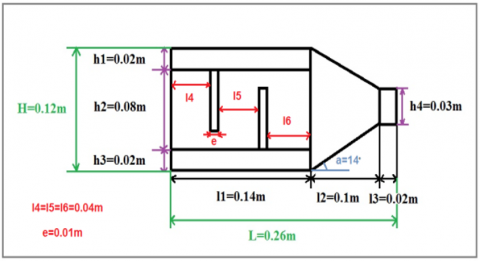Figure 1. The studied geometry

The problem is as mentioned previously in the analysis and the study of an air flow of forced convection in turbulent behavior passes through a rectangular channel length L provided with fins and transverse baffles, the Figure 1 represent the geometry studied.

C. Boundary conditions

Summarizes the boundary conditions in the following table:

-The temperature inside the channel: Ti = 278 K.

-Channel entry (the same for the three entries): Te=303 K Ve=0.1 m/s

- High of channel: Q=650 W/m².

- Channel output (only one output): Ts=295 K Ps=1 at m.

- Lower channel: T=300 K.

3. Results and Interpretations

Simulation and interpretation of numerical results obtained in the studied configurations. We present the validation with the work of the bibliography in the Figure 2.

## 2.pngFigure 2. The boundary conditions

1. Validation of results

From the Figure 3 we can see that there is a good concordance between the axial velocities.

## 3.png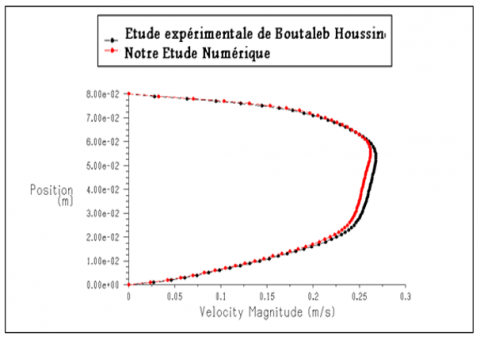Figure 3. Validation of the numerical simulation with experimental results 

1. Discussion
• The velocity field

In the regions between the tip of the baffle and the channel walls, the velocity is increased, due to the narrowing of the section of the passage of the fluid.

The highest values of the speed appear near the top of the channel with an acceleration process that begins just after the second chicane as shown in the Figure 4.

## 4.png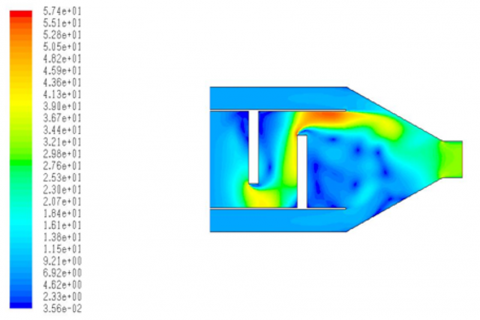Figure 4. Contours de Velocity

## 5.png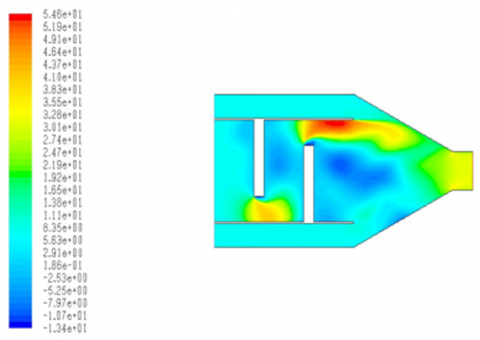Figure 5. Contour of the velocity U

The Figure 5 turned out to the contour of the velocity longitudinal U.

The Figure 6 represent the contour of the velocity transversal V.

## 6.png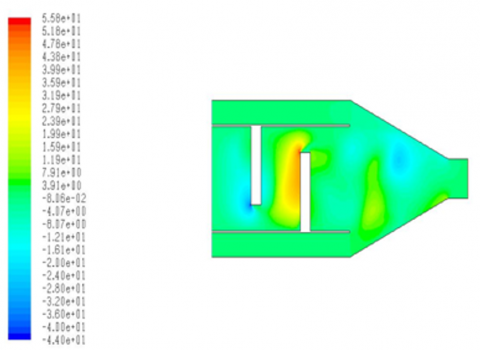Figure 6. Contour of the velocity V

• The Temperature field

## 7.png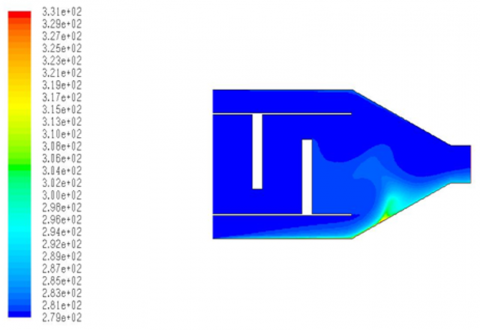Figure 7. Contour of the temperature

The Figure 7 notes that the temperature just downstream becomes important in the second chicane.

• Velocity profile

## 8.pngFigure 8. Profile of the velocity in the second part of canal

The Figure 8 represent the velocity in the second part of canal (x=0.16 m, x=0.18 m, x=0.22 m and x=0.2 m), we note that the velocity increases because the divergence of canal.

The Figure 9 shown the profile of the velocity in the first part of canal (x=0.02 m, x=0.07 m and x=0.12 m), we note that the velocity begins to increase downstream of the first baffle and takes a large value downstream of the second baffle.

## 9.png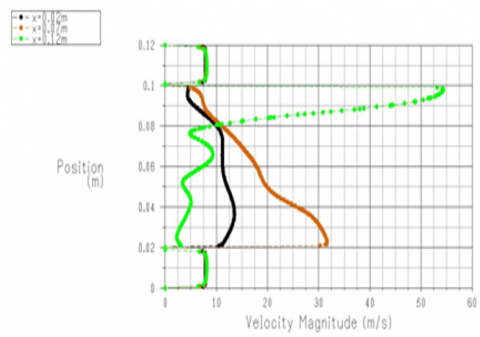Figure 9. Profile of the velocity in the first part of canal

• Temperature profile

## 10.png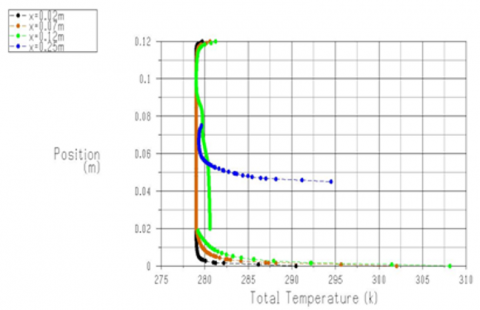Figure 10. Profile of the Temperature at the sections x=0.02 m, x=0.07 m, x=0.12 m and x=0.25 m

The Figure 10 shows a drop in temperature in the sections x=0.02 m, x=0.07 m, x=0.12 m and x=0.25 m.

The hottest areas are located near the walls and the ends of the baffles.

We also note that the total temperature values are substantially higher in the vicinity of the baffles especially in areas downstream of the lower baffles.

The air temperature increases as the fluid is once again in contact with the baffles, and this is repeated in an analogous manner from one cell to another.

4. Conclusions

In this work, I study the simulation of the flow in a solar collector with a rectangular baffle for air. It is a rather disorganized complex flow structure, particularly near the walls of the channel where the fluid is diverted to the upper and lower walls at high velocities. A strong vortex downstream of each baffle is observed.

This flow structure will considerably affect the distribution of the temperature field and allow better mixing of the fluid. The temperature field also shows a drop in temperature in the areas between the top of each baffle and the walls of the canal. Where the hottest areas are mainly located near the walls and the extremities of the baffles. It has been found that an inverse proportionality between the increase in axial velocity and the decrease in overall temperature in each cross section of the channel, this means that the temperature gradient at the heated walls decreases with increasing flow of velocity.

References

 Bemar, C., Durst, F., Mceligot, D.M. (1984). Numerical study of fluid flow and heat transfer in a parallel plate channel with baffles. Journal of Heat Transfer, 106-743.

 Cheng, C.H., Huang, W.H. (1991). Numerical prediction for laminar forced convection in parallel-plate channels with transverse fin arrays. International Journal of Heat and Mass Transfer, 34(11): 2739-2749. https://doi.org/10.1016/0017-9310(91)90232-4

 Hwang, R.R., Chow, Y.C., Peng, Y.F. (1999). Numerical study of turbulent flow over-tow dimensional surface-mounted ribs in a channel. International Journal for Numerical Methods in Fluids, 37(4): 767-785.https://doi.org/10.1002/(SICI)1097-0363(19991030)31:4<767::AID-FLD902>3.0.CO;2-A

 Saim, R., Abboudi, S., Benyoucef, B., Azzi, A. (2007). Analyse numérique de la convection force turbulente dans les tubes munis des chicanes transversales. 13 èmes Journées Internationales de Thermique, Albi, pp. 1-5, France du 28 au 30 Août 2007.

 Aliane, K., Amraoui, M. (2013). Etude numérique d’un capteur solaire plan à air ayant une rugosité rectangulaire. Revue des Energies Renouvelables, 16(1): 129-141.

 Youcef-Ali, S. (2005). Study and optimization of the thermal performances of the offset rectangular plate fin absorber plates, with various glazing. Renewable Energy, 30: 271-280.

 Oudrane, A., Aour, B., Zeghmati, B., Chesneau, X., Hamouda, M. (2017). Etude et calcul de bilan de la densité du flux solaire pour un simple habitat dans la région d’Adrar. Revue des Energies Renouvelables, 20(1): 51-60.

 Ghodbane, M., Boumeddane, B., Moummi, N., Largot, S., Berkane, H. (2017). Study and numerical simulation of solar system for air heating. Journal of Fundamental and Applied Sciences, 8(1): 41-60.

 Ghodbane, M., Boumeddane, B., Largot, S., Heniat, N. (2015). Simulation Numérique d’un Concentrateur Cylindro-Parabolique en El Oued, Algérie.International Journal of Scientific Research & Engineering Technology (IJSET), 3(2): 68-74.

 Nacereddine, R. (2007). Conception optimisée d’un échangeur à un rang de tubes ailettés : minimisation de la dépense énergétique de fonctionnement. Département Energétique Industrielle, Ecole des Mines de Douai 941, rue Charles Bourseul - BP 10838 - 59508 DOUAI Cedex.

 Younsi, Z. (2009). Étude expérimentale d'un mur trombe-composite à échelle réduite intégrant des materiaux à changement de phase. Faculté des Sciences Appliquées de l'Université d'Artois, Department of applied sciences – Université du Québec à Chicoutimi, Canada, IXème Colloque Interuniversitaire Franco-Québécois sur la Thermique des Systèmes, Lille.

 Hami, K. (2010). Modélisation d’un système de chauffage passif dans la région de Béchar K. Revue des Energies Renouvelables, 13(2): 355-368.

 Bourhaleb, H. (1987). Etude et expérimentation dune chaine énergétique solaire avec capteur à air, stockage thermique souterrain et récupération par pompe à chaleur. Thèse de doctorat 3éme cycles en Energétique, Université HAINAVT Cambresis.